Name:    Editing Questions #18

Multiple Choice
Identify the letter of the choice that is incorrect. Problems could include grammar (e.g. subject-verb agreement), usage, word choice, spelling, punctuation and changes in tense. Choose “D” if no problem exists.

Be aware that all of the sample sentences are treated as parts of one whole article.
____________________

1.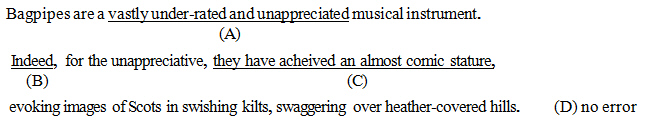a. A b. B c. C d. D

2.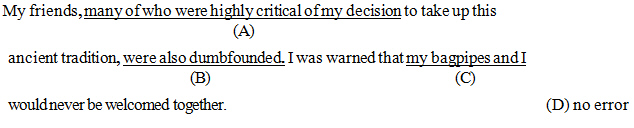a. A b. B c. C d. D

3.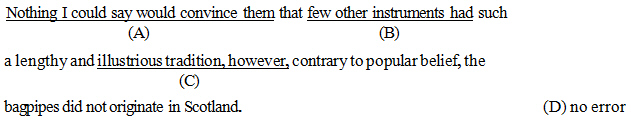a. A b. B c. C d. D

4.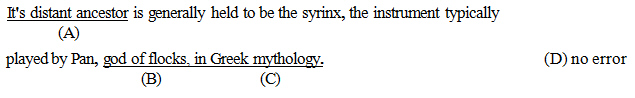a. A b. B c. C d. D

5.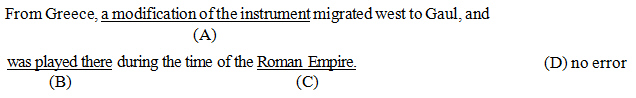a. A b. B c. C d. D

6.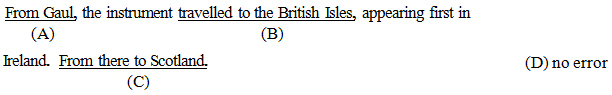a. A b. B c. C d. D

7.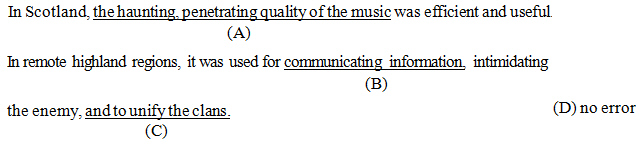a. A b. B c. C d. D

8.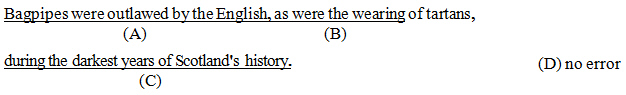a. A b. B c. C d. D

9.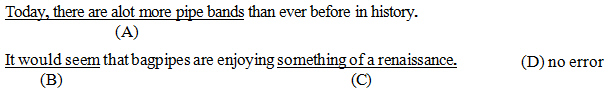a. A b. B c. C d. D

10.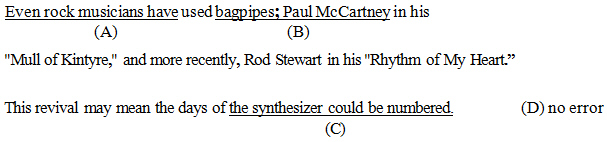a. A b. B c. C d. D